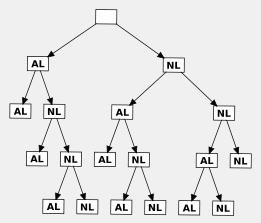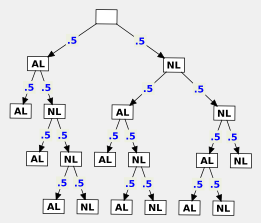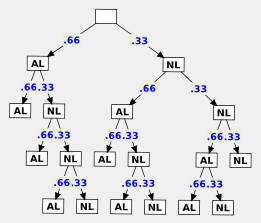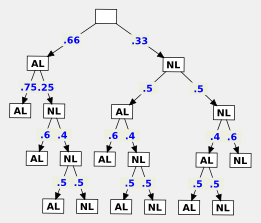# the world series

A common probability problem you might find in a text book (that's where I found it) is judging the probability of the outcome of a partially played world series. The scenario is this: Three games of a seven game world series have been played. The American League is up two games to one, over the National League. From this setup, we can create a variety of questions: How many different possible outcomes are there for the remaining games in the series? What is the probability that either the American League or the National League will win the series? What is the probability of the series going an additional two games, or three games, or four games?The question that got me thinking was: 'What is the probability that the series will run to seven games?' To begin with, we can enumerate all of the possible outcomes of each remaining game in tree form. Each labeled node of the tree represents a possible winner of a successive game. By counting the paths through this tree, or alternatively the leaf nodes, we can see that there are ten possible sequences of events that can follow from a 2-1 standing in a seven game series.

Six of these ten outcomes requires the play of seven games. It may be tempting then to say that the probability that the series will extend to seven games is 0.6. This, however isn't the case. First, there isn't a single event, of which there are 10 possible outcomes. There is instead possibly two events (in the case that the AL wins the next two games), or three events (in the case that the NL wins the next three games), or perhaps four events. Each of these events has two possible outcomes: The NL wins, or the AL wins. Secondly, none of these events have a probability assigned to them.We can take a first stab at an answer by recognizing that there is a sequence of events, and assigning a random probability to each: 0.5. Then calculating the probability of each sequence. There are ten possible sequences. Assuming a random probability of the winner of each game, each of the paths through the tree which follow to four additional games has a probability of 0.0625. There are six of these paths, which added together gives a probability of .375 that the series will extend to seven games.

I think this answer qualifies as 'correct' given the assumption that the outcome of each game is random. Though, I don't think it's particularly accurate. The outcome of each game is likely not random. One team is surely better than the other. This should be reflected in the outcome of their past meetings. And, luckily, we have a sample of their past meetings in the current series standings. The American league is up two games to one.Based on this (admittedly small) selection of data we can say that the probability of the American League team winning a game against the the National League team is 2/3,or .66, and the probability of the National League team winning such a matchup is 1/3, or .33. If we insert these values into the probability tree, each path that leads to four additional games has the probability of either 0.049 or 0.025. By adding the probabilities of these six paths together, we arrive at the figure 0.222. A slightly lower probability that the series will extend to seven games.This answer, as well, seems to be reasonably 'correct'. But, given the data we have, I think there is a, yet, more accurate way to calculate the probabilities of the outcome of each game. We assigned a single probability based on the outcome of three games to game four, five, six and seven. Wouldn't the probability of the outcome of game seven be more accurately estimated, if it were based upon the results of six previous games, rather than three? And likewise, the probability of the outcome of game six estimated on the results of the five previous games? If we apply those figures to the probability tree, the resulting probability that the series will run to seven games is 0.2.

Given that all we know is the current standings in the series, I think that's the most accurate answer. In a 'real' situation, the probability is surely affected by myriad other factors: home and away games, injuries, weather, a much more thorough game history, etc. I'd be interested to know if I'm wildly wrong, or if there is a better way to figure this, given the constraints of the problem.